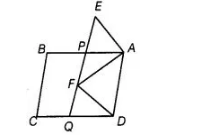# In figure, ABCD and AEFD are two`
Question:

In figure, ABCD and AEFD are two parallelograms. Prove that ar (APEA) = ar(AQFO).Solution:

Given, ABCD and AEFD are two parallelograms.

To prove ar (APEA) = ar (AQFD)

AP || DQ [since, in parallelogram ABCD, AB || CD ] and PQ || AD [since, in parallelogram AEFD, FE || AD]

Then, quadrilateral PQDA is a parallelogram.

Also, parallelogram PQDA and AEFD are on the same base AD and between the same parallels AD and EQ.

ar (parallelogram PQDA) = ar (parallelogram AEFD)

On subtracting ar (quadrilateral APFD) from both sides, we get

ar (parallelogram PQDA)- ar (quadrilateral APFD)

= ar (parallelogram AEFD) – ar (quadrilateral APFD) => ar (AQFD) = ar (APEA) Hence proved.